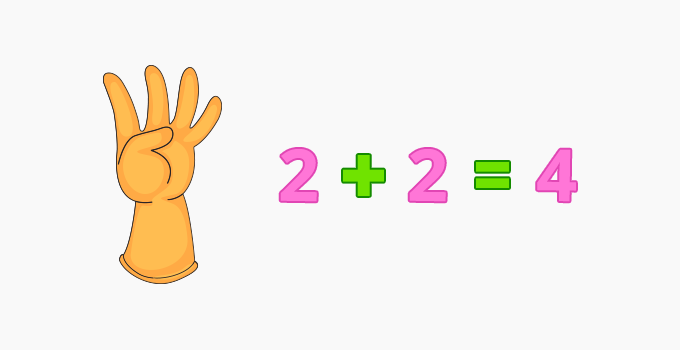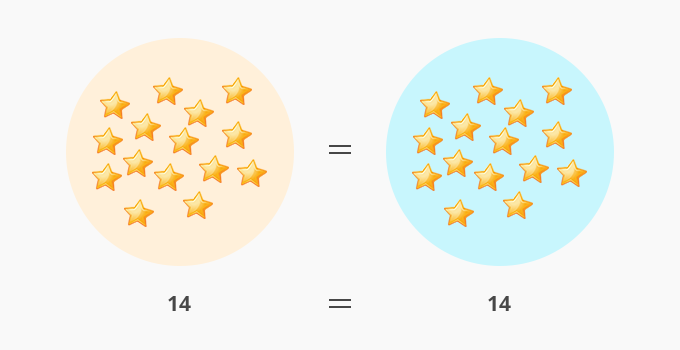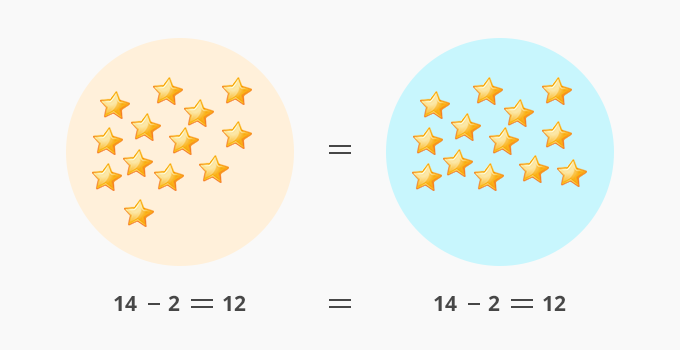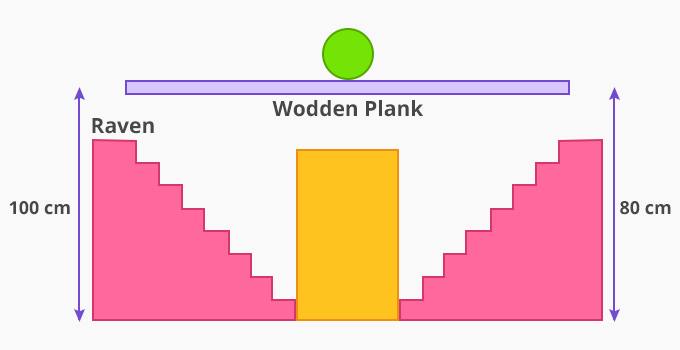# Subtraction Property Of Equality - Definition with Examples

The Complete K-5 Math Learning Program Built for Your Child

• 40 Million Kids

Loved by kids and parent worldwide

• 50,000 Schools

Trusted by teachers across schools

• Comprehensive Curriculum

Aligned to Common Core

## Subtraction Property of Equality

Equations are expressions having two equal parts on each side of an ‘equal to sign’.## Subtraction property of equality

Subtraction property of equality refers to balancing an equation by using the same mathematical operation on both sides.

For instance: We have 2 circles with the same number of stars.Stars in Circle 1 = Stars in Circle 2

= 14 = 14

If we take away 2 stars from Circle 1 then, to balance this equation in both the circles we need to take away 2 stars from the other circle also.So, we can write an equation:

if a = b

then, a - c = b - c

Example 1:John wishes to fly using10 balloons. He has 10 in one hand and 10 in another. As he sets to fly, a bird bursts 3 balloons on one side, making him lose balance. How many balloons should he let go to regain his balance?

Solution:

To solve this question, we have to use the subtraction property of equality.

Initially, the person is holding 10 balloons in both of his hands.

10 = 10

Then, 3 balloons were destroyed by a bird, resulting in a loss of balance.

10 - 3 = 10 ⇒ 7 = 10 [Both sides are not balanced which makes it incorrect]

To balance the other side, John lets go of 3 balloons.

7 = 10 - 3 ⇒ 7 = 7 [Equation is balanced as both sides are equal]

Example 2: Raven and Maddy are playing a game of balancing a ball on a wooden plank. Raven says, “I am going 20 cm down”. To keep the ball balanced on the wooden plank, where should Maddy go?Solution:

To solve this question, we have to use the subtraction property of equality.

Initially, the ball is balanced as Raven and Maddy are at the same height.

70 cm =70 cm

Now, Raven is going 20 cm down. To keep the ball balanced Maddy must also step down by 20 cm  70 - 20 = 70 - 20 ⇒ 50 cm = 50 cm

 Fun Facts Addition and subtraction are inverse operations.

Won Numerous Awards & Honors